# Create Mathematical Documentswith MathPad for Mac and / or iOS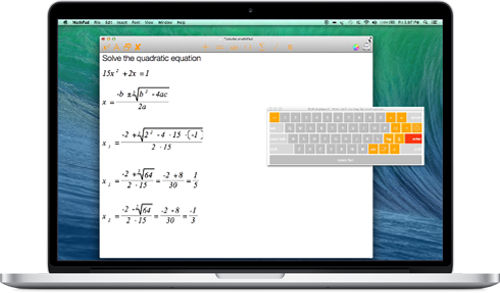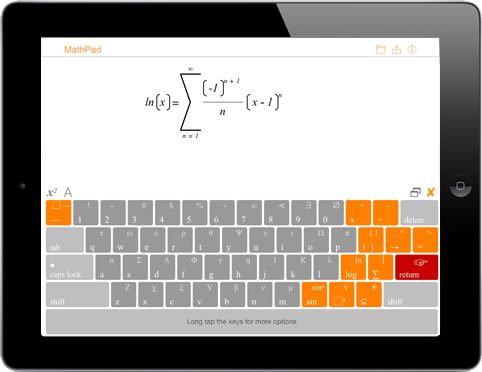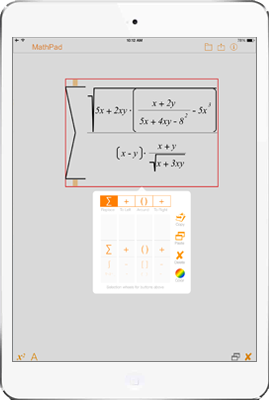# Creative, Useful Apps for Mac and iOS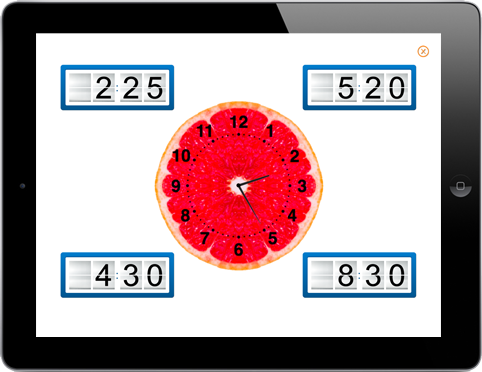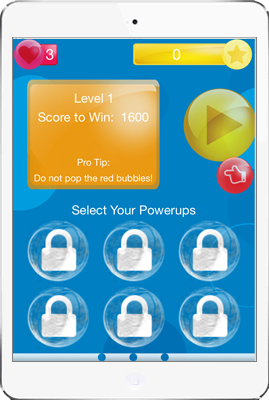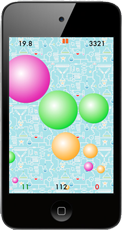### For iOS

##### Learn how to use MathPad to solve a quadratic equation

MathPad allows you to create documents with mathematical expressions using a specially designed mathematical keyboard.

Learn how to use MathPad to solve a quadratic equation.

##### MathPad – Shapes

Create documents with mathematical expressions on Mac or iPad

Geometric shapes can now be inserted into a document

In MathPad for Mac, use the Insert menu to add shapes

In MathPad for iOS, use the add menu to insert shapes

The notations included in the shapes are editable

### For macOS

##### MathPad EDU – Tutorial

MathPad allows you to create documents with mathematical expressions using a specially designed mathematical keyboard.

When typing expressions, the physical keyboard becomes a mathematical keyboard.